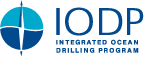IODP Proceedings    Volume contents     Searchdoi:10.2204/iodp.proc.337.202.2017

## Results

The permeability determinations are listed in Table T2. Coefficients of permeability for the two coal samples are 1.31 × 10–9 cm/s and 1.23 × 10–9 cm/s at 40 MPa confining pressure and 6.72 × 10–11 cm/s and 6.38 × 10–11 cm/s at 50 MPa confining pressure, respectively. The intrinsic permeabilities of the two samples were 7.38 × 10–19 m2 and 6.93 × 10–19 m2 at 40 MPa confining pressure and 3.80 × 10–20 m2 and 3.61 × 10–20 m2 at 50 MPa confining pressure, respectively.

The results of the grain-size measurements are shown in Figure F2 and Table T3. The sand size fraction (passing through sieves coarser than 75 µm) is 88.5 wt%, and the silt and clay size fraction (<75 µm) is 11.5 wt%. The estimated porosity was 40.5%. For comparison, the estimated porosity is plotted with onboard measurements of core samples in Figure F3.